# Percentage Questions For Class 7

Percentage Questions For Class 7, deals with various concepts which are as under:-

• Find sum of money when a Percentage of such sum is given
• Finding actual quantity from Percentage value
• Find what percentage of a given quantity is another term
• Finding a number when its decreased percentage is given
• Finding a number when its increased percentage is given

#### Percentage Questions For Class 7 – Find sum of money when a Percentage of such sum is given

Question 1

A man has a certain unknown sum of money, 60 % of that sum is ₹ 4800. What is the sum of money?

Explanation:

The problem can be solved using linear equation.
Let the unknown sum of money be ₹ a
We know, that 60 % of ₹ a = ₹ 4800

60 % x a = ₹ ( 4800 )
60/100 x a = ₹ ( 4800 )

a = ₹ (4800 x 100)/60

= ₹ 8000
Therefore, the unknown sum of money available with the man is ₹ 8000

#### Percentage Questions For Class 7 – Finding actual quantity from Percentage value

Question 2

Find 71 % of 3600 metres

Explanation:

Step 1 – Convert the Percentage into Fraction
i.e 71 % = 71/100

Step 2 – Multiply the Fraction with given quantity.
i.e, ( 71/100 x 3600 ) metres = 2556 metres

Hence, 71 % of 3600 metres = 2556 metres

#### Percentage Questions For Class 7 – Find what percentage of a given quantity is another term

Question 3

What percent of 60 m is 9 m ?

Explanation:

Let a % of 60 m = 9 m

Step 1 – Convert the Percentage into Fraction
i.e a % = a/100

Step 2 – Multiply the Fraction with given quantity.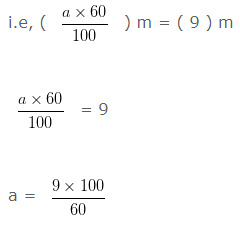a = 15
Hence, 9 m is 15 % of 60 m

Question 4

What percent of 80 kg is 28 kg ?

Explanation:

Let a % of 80 kg = 28 kg

Step 1 – Convert the Percentage into Fraction
i.e a % = a/100

Step 2 – Multiply the Fraction with given quantity.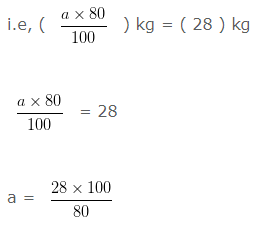a = 35

Hence, 28 kg is 35 % of 80 kg

Question 5

What percent of 30 litres is 6 litres ?

Explanation:

Let a % of 30 litres = 6 litres
Step 1 – Convert the Percentage into Fraction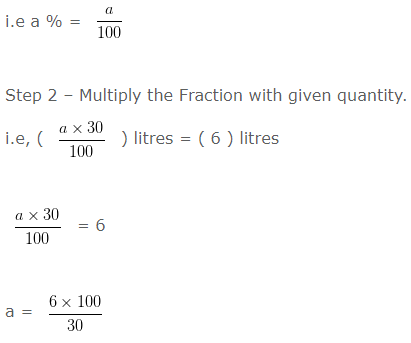a = 20
Hence, 6 litres is 20 % of 30 litres

#### Percentage Questions For Class 7 – Finding a number when its decreased percentage is given

Question 6

A number when decreased by  10 % gives 45 . The number is?

Explanation:

Here we take the help of linear equation. First we will form the equation.
Let the number be  ‘a’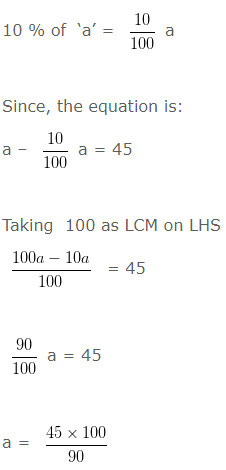a = 50
Hence, the number is 50

#### Percentage Questions For Class 7 – Finding a number when its increased percentage is given

Question 7

A number, when increased by 40 % gives 84 . The number is?

Explanation:

The problem can be solved using linear equation.
Let the number be a’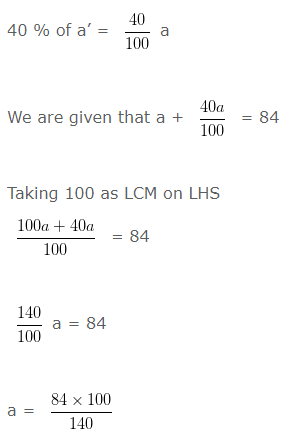a = 60
Hence, the number is 60

### 3 thoughts on “Percentage Questions For Class 7”

1.••